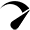# How to Calculate Solar Panel Battery and Inverter?

## Step 1:- Home electrical load calculation?

4 Led Light    = 4×25 = 100W

2 fan              = 2×80 = 160W

1 Tube Light = 1×40 = 40W

1 Led TV        = 1×50 = 50W

## Step 2:- Inverter Battery

Consider – 500W

However, the Recommendation is 800-1000W best

Battery DC Volt = 12V

DC current required

P = V x I

I=P/V

350W AC Load …DC current required (I) =29.17=30A

## Step 3:- Battery Size Selection in AH

Formula:-W x H/V

Where: – W= Total load (350w)

H= Backup time in hours (8)

V= Battery voltage (12v)

=350X8/12=233AH

Battery Standard: – 250AH

Battery Select: – 250AH

Battery Charging Current

We will calculate the charging current for the 250AH battery. As we know the charging current should be 10% of the AH rating of the Battery.

Therefore

Charging current for 250AH Battery

= 250X10%

= 25A

## Step 4:-Solar Panel Selection?

Solar panel Current =Battery charging current + Home load current

= 25+30

= 55 A

Solar plate power

Formula = V X I

= 12 X 55

= 660W

1. Of Solar panels

340W Home load (AC) = 660W Solar panel required DC

The 660w Solar panel below is available in the market.

1. 125w
2. 180w
3. 375w
4. 440w

No. of solar plates =Total solar plates watt/single panel watts

=660/180

= 3.66 Approx. (4)

No. of solar plates:-4 panels (4x 180w)Optimized by Seraphinite Accelerator